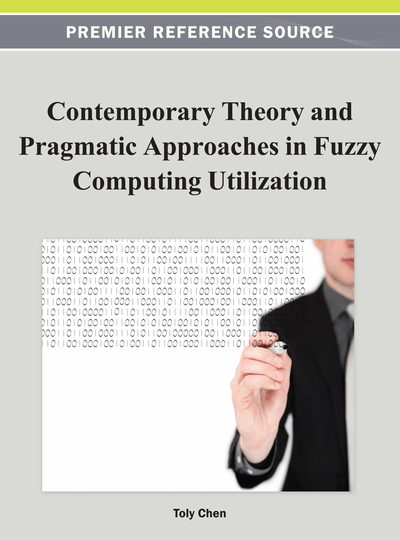# Fuzzy Cluster Validation Based on Fuzzy PCA-Guided Procedure

K. Honda (Osaka Prefecture University, Japan), A. Notsu (Osaka Prefecture University, Japan), T. Matsui (Osaka Prefecture University, Japan) and H. Ichihashi (Osaka Prefecture University, Japan)
DOI: 10.4018/978-1-4666-1870-1.ch002
Available
\$37.50
No Current Special Offers

## Abstract

Cluster validation is an important issue in fuzzy clustering research and many validity measures, most of which are motivated by intuitive justification considering geometrical features, have been developed. This paper proposes a new validation approach, which evaluates the validity degree of cluster partitions from the view point of the optimality of objective functions in FCM-type clustering. This approach makes it possible to evaluate the validity degree of robust cluster partitions, in which geometrical features are not available because of their possibilistic natures.
Chapter Preview
Top

## Introduction

Fuzzy clustering (or fuzzy cluster analysis) is a most active research area in the fuzzy systems field. The fuzzy c-means (FCM) algorithm (Bezdek, 1981) and its variants (Höppner et al., 1999; Miyamoto et al., 2008) have been proved to be useful for data summarization. In the FCM-type clustering models, the objective function is given as the fuzzy membership-weighted inner-cluster errors between data points and cluster prototypes. Although the algorithms are designed well for finding local optimal solutions based on the iterative optimization scheme, they often derive several different local solutions in the multi-starting strategy. Additionally, the optimal cluster number is not known a priori. Therefore, we need the validation measure for selecting the optimal cluster partition from multiple solutions.

Many validation measures have been proposed, some of which were designed for finding compact and separate clusters from the view point of intuitive geometrical features. Xie-Beni index (Xie & Beni, 1987) and other indices based on similar concepts (Dunn, 1974, Fukuyama & Sugeno, 1989) measures the cluster separateness using distances among cluster centers. Another approach considers cluster overlapping without using prototypes (Bezdek, 1981; Kim et al., 2003). Although these measures have been intuitively justified, it is not necessarily guaranteed that the validation measures really suit the evaluation of ‘local optima’ of objective functions. So, Rankler (2007) considered the pareto optimal solutions in multi-objective problems of objective functions and validation measures. In this paper, the cluster validity is discussed considering only the optimality of objective functions.

Another topic considered in this paper is noise rejection mechanism in the FCM-type clustering. Noise fuzzy clustering (Davé, 1991) uses an additional “noise cluster” so that noise samples are dumped into it. Masulli and Rovetta (2006) proposed a technique for soft transition from the conventional FCM constraint to a robust situation where noise samples are rejected (or ignored). Although noise rejection mechanisms are useful for obtaining cluster structures for contaminated data, they also create difficulties when we apply conventional validity measures designed for fuzzy clustering.

In this paper, a new cluster validation approach is considered based on principal component analysis (PCA)-guided procedures. The PCA-guided k-means (Ding & He, 2004a) is a deterministic method for finding the optimal k-means partition, in which a relaxed cluster indicator is estimated from a rotated principal component score matrix, though the rotation matrix cannot be explicitly estimated. Honda et al. (2010) introduced the noise rejection mechanism and proposed fuzzy PCA-guided robust k-means (FPR k-means). Because these PCA-guided procedures can find the optimal cluster structure considering only the optimality of objective functions, we can validate candidate cluster partitions if we estimate the rotation matrix for reconstructing the cluster indicators. In the proposed validation approach, a candidate for the pseudo-optimal indicator is first reconstructed by Procrustean transformation (Procrustean rotation) and a fair deviation from the pseudo-optimal solution is calculated for the current partition. Then, the cluster partition having least deviation is selected.

The remainder of the article is organized as follows. The next section describes some related works. The following section outlines the proposed validation approach. Experimental results are then shown. The final section outlines a summary and the conclusions.

## Complete Chapter List

Search this Book:
Reset